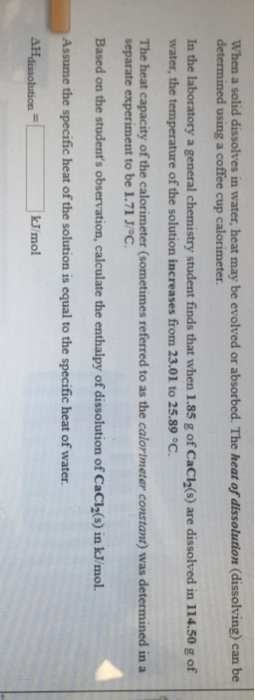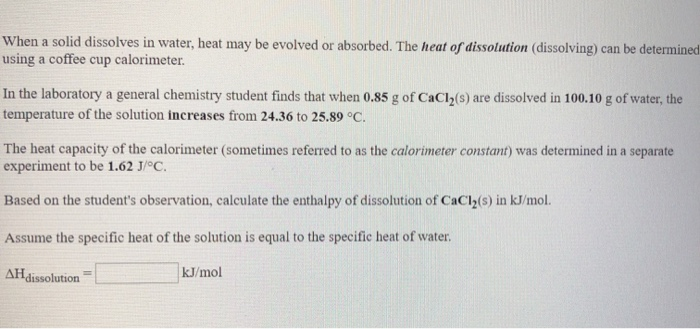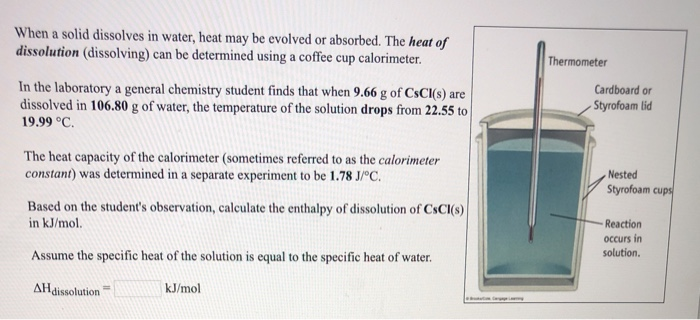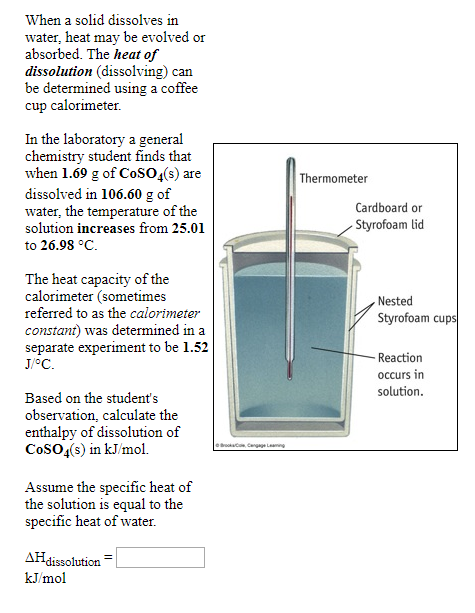# When a solid dissolves in water, heat may be evolved or absorbed. The heat of dissolution...When a solid dissolves in water, heat may be evolved or absorbed. The heat of dissolution (dissolving) can be determined using a coffee cup calorimeter In the laboratory a general chemistry student finds that when 1.85 g of CaCl2(s) are dissolved in 114.50 g of water, the temperature of the solution increases from 23.01 to 25.89 °C The heat capacity of the calorimeter (sometimes referred to as the calortmeter constant) was determined in a separate experiment to be 1.71 J/OC Based on the student's observation, calculate the enthalpy of dissolution of CaCl2(s) in kJ/mol. Assume the specific heat of the solution is equal to the specific heat of water. kJ/mol

This Homework Help Question: "When a solid dissolves in water, heat may be evolved or absorbed. The heat of dissolution..." No answers yet.

We need 10 more requests to produce the answer to this homework help question. Share with your friends to get the answer faster!

0 /10 have requested the answer to this homework help question.

Once 10 people have made a request, the answer to this question will be available in 1-2 days.
All students who have requested the answer will be notified once they are available.

#### Earn Coin

Coins can be redeemed for fabulous gifts.

Similar Homework Help Questions
• ### please help When a solid dissolves in water, heat may be evolved or absorbed. The heat...please help When a solid dissolves in water, heat may be evolved or absorbed. The heat of dissolution (dissolving) can be determined using a coffee cup calorimeter. In the laboratory a general chemistry student finds that when 0.85 g of CaCl2(s) are dissolved in 100.10 g of water, the temperature of the solution increases from 24.36 to 25.89 °C. The heat capacity of the calorimeter (sometimes referred to as the calorimeter constant) was determined in a separate experiment to be...

• ### When a solid dissolves in water, heat may be evolved or absorbed. The heat of dissolution...When a solid dissolves in water, heat may be evolved or absorbed. The heat of dissolution (dissolving) can be determined using a coffee cup calorimeter. Thermometer In the laboratory a general chemistry student finds that when 9.66 g of CSCI(S) are dissolved in 106.80 g of water, the temperature of the solution drops from 22.55 to 19.99 °C. Cardboard or Styrofoam lid The heat capacity of the calorimeter (sometimes referred to as the calorimeter constant) was determined in a separate...

• ### a. When a solid dissolves in water, heat may be evolved or absorbed. The heat of...

a. When a solid dissolves in water, heat may be evolved or absorbed. The heat of dissolution (dissolving) can be determined using a coffee cup calorimeter. In the laboratory a general chemistry student finds that when 16.11 g of BaCl2(s) are dissolved in 118.70 g of water, the temperature of the solution increases from 22.98 to 25.41 °C. The heat capacity of the calorimeter (sometimes referred to as the calorimeter constant) was determined in a separate experiment to be 1.71...

• ### Part A: When a solid dissolves in water, heat may be evolved or absorbed. The heat of dissolution (dissolving) can be de...

Part A: When a solid dissolves in water, heat may be evolved or absorbed. The heat of dissolution (dissolving) can be determined using a coffee cup calorimeter. In the laboratory a general chemistry student finds that when 2.79 g of NH4Cl(s) are dissolved in 106.00 g of water, the temperature of the solution drops from 23.17 to 21.34 °C. The heat capacity of the calorimeter (sometimes referred to as the calorimeter constant) was determined in a separate experiment to be...

• ### When a solid dissolves in water, heat may be evolved or absorbed. The heat of dissolution...

When a solid dissolves in water, heat may be evolved or absorbed. The heat of dissolution (dissolving) can be determined using a coffee cup calorimeter. In the laboratory a general chemistry student finds that when 9.35 g of K2SO4(s) are dissolved in 104.60 g of water, the temperature of the solution drops from 24.91 to 21.62 °C. The heat capacity of the calorimeter (sometimes referred to as the calorimeter constant) was determined in a separate experiment to be 1.77 J/°C....

• ### When a solid dissolves in water, heat may be evolved or absorbed. The heat of dissolution...

When a solid dissolves in water, heat may be evolved or absorbed. The heat of dissolution (dissolving) can be determined using a coffee cup calorimeter. In the laboratory a general chemistry student finds that when 2.63 g of KClO4(s) are dissolved in 102.50 g of water, the temperature of the solution drops from 24.36 to 21.97 °C. The heat capacity of the calorimeter (sometimes referred to as the calorimeter constant) was determined in a separate experiment to be 1.58 J/°C....

• ### When a solid dissolves in water, heat may be evolved or absorbed. The heat of dissolution...

When a solid dissolves in water, heat may be evolved or absorbed. The heat of dissolution (dissolving) can be determined using a coffee cup calorimeter. In the laboratory a general chemistry student finds that when 18.08 g of BaBr2(s) are dissolved in 111.20 g of water, the temperature of the solution increases from 24.15 to 26.91 °C. The heat capacity of the calorimeter (sometimes referred to as the calorimeter constant) was determined in a separate experiment to be 1.81 J/°C....

• ### When a solid dissolves in water, heat may be evolved or absorbed. The heat of dissolution...

When a solid dissolves in water, heat may be evolved or absorbed. The heat of dissolution (dissolving) can be determined using a coffee cup calorimeter. In the laboratory a general chemistry student finds that when 4.82 g of CsClO4(s) are dissolved in 118.30 g of water, the temperature of the solution drops from 23.64 to 21.14 °C. The heat capacity of the calorimeter (sometimes referred to as the calorimeter constant) was determined in a separate experiment to be 1.82 J/°C....

• ### When a solid dissolves in water, heat may be evolved or absorbed. The heat of dissolution...

When a solid dissolves in water, heat may be evolved or absorbed. The heat of dissolution (dissolving) can be determined using a coffee cup calorimeter. In the laboratory a general chemistry student finds that when 5.34 g of KBr(s) are dissolved in 112.60 g of water, the temperature of the solution drops from 24.33 to 22.29 °C. The heat capacity of the calorimeter (sometimes referred to as the calorimeter constant) was determined in a separate experiment to be1.82 J/°C. Based...

• ### When a solid dissolves in water, heat may be evolved or absorbed. The heat of dissolution...When a solid dissolves in water, heat may be evolved or absorbed. The heat of dissolution (dissolving) can be determined using a coffee cup calorimeter. Thermometer In the laboratory a general chemistry student finds that when 1.69 g of CoSO4(s) are dissolved in 106.60 g of water, the temperature of the solution increases from 25.01 to 26.98 °C. Cardboard or Styrofoam lid The heat capacity of the calorimeter (sometimes referred to as the calorimeter constant) was determined in a separate...